## Overview

Recall that the job of the scanner is to translate the sequence of characters that is the input to the compiler to a corresponding sequence of tokens. In particular, each time the scanner is called it should find the longest sequence of characters in the input, starting with the current character, that corresponds to a token, and should return that token.

It is possible to write a scanner from scratch, but a more efficient and less error-prone approach is to use a scanner generator like lex or flex (which produce C code), or JLex (which produces Java code). The input to a scanner generator includes one regular expression for each token (and for each construct that must be recognized and ignored, such as whitespace and comments). Therefore, to use a scanner generator you need to understand regular expressions. To understand how the scanner generator produces code that correctly recognizes the strings defined by the regular expressions, you need to understand finite-state machines (FSMs).

## Finite-State Machines

A finite-state machine is similar to a compiler in that:

• A compiler recognizes legal programs in some (source) language.
• A finite-state machine recognizes legal strings in some language.
In both cases, the input (the program or the string) is a sequence of characters.

### Example: Pascal Identifiers

Here's an example of a finite-state-machine that recognizes Pascal identifiers (sequences of one or more letters or digits, starting with a letter):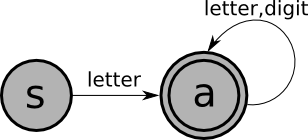In this picture:

• Nodes are states.
• Edges (arrows) are transitions. Each edge should be labeled with a single character. In this example, we've used a single edge labeled "letter" to stand for 52 edges labeled 'a', 'b', ..., 'z', 'A', ..., 'Z'. (Similarly, the label "letter,digit" stands for 62 edges labeled 'a',...'Z','0',...'9'.)
• S is the start state; every FSM has exactly one (a standard convention is to label the start state "S").
• A is a final state. By convention, final states are drawn using a double circle, and non-final states are drawn using single circles. A FSM may have more than one final state.
A FSM is applied to an input (a sequence of characters). It either accepts or rejects that input. Here's how the FSM works:
• The FSM starts in its start state.
• If there is a edge out of the current state whose label matches the current input character, then the FSM moves to the state pointed to by that edge, and "consumes" that character; otherwise, it gets stuck.
• The finite-state machine stops when it gets stuck or when it has consumed all of the input characters.

An input string is accepted by a FSM if:

• The entire string is consumed (the machine did not get stuck), and
• the machine ends in a final state.
The language defined by a FSM is the set of strings accepted by the FSM.

The following strings are in the language of the FSM shown above:

• x
• tmp2
• XyZzy
• position27
The following strings are not in the language of the FSM shown above:
• 123
• a?
• 13apples

TEST YOURSELF #1

Write a finite-state machine that accepts e-mail addresses, defined as follows:

• a sequence of one or more letters and/or digits,
• followed by an at-sign,
• followed by one or more letters,
• followed by zero or more extensions.
• An extension is a dot followed by one or more letters.

solution

## Example: Integer Literals

The following is a finite-state machine that accepts integer literals with an optional + or - sign: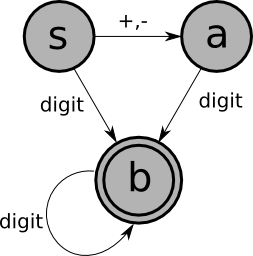## Formal Definition

An FSM is a 5-tuple: $(Q,\Sigma,\delta,q,F)$

• $Q$ is a finite set of states ($\{\mathrm{S,A,B}\}$ in the above example).
• $\Sigma$ (an uppercase sigma) is the alphabet of the machine, a finite set of characters that label the edges ($\{+,-,0,1,...,9\}$ in the above example).
• $q$ is the start state, an element of $Q$ ($\mathrm{S}$ in the above example).
• $F$ is the set of final states, a subset of $Q$ ({B} in the above example).
• $\delta$ is the state transition relation: $Q \times \Sigma \rightarrow Q$ (i.e., it is a function that takes two arguments -- a state in $Q$ and a character in $\Sigma$ -- and returns a state in $Q$).

Here's a definition of $\delta$ for the above example, using a state transition table:

 + - $\mathrm{digit}$ $S$ $A$ $A$ $B$ $A$ $B$ $B$ $B$

## Deterministic and Non-Deterministic FSMs

There are two kinds of FSM:

1. Deterministic:
• No state has more than one outgoing edge with the same label.
2. Non-Deterministic:
• States may have more than one outgoing edge with same label.
• Edges may be labeled with $\varepsilon$ (epsilon), the empty string. The FSM can take an $\varepsilon$-transition without looking at the current input character.

Example

Here is a non-deterministic finite-state machine that recognizes the same language as the second example deterministic FSM above (the language of integer literals with an optional sign):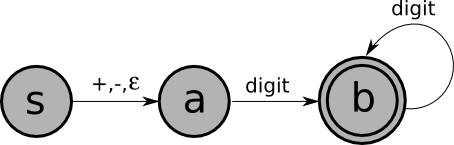Sometimes, non-deterministic machines are simpler than deterministic ones, though not in this example.

A string is accepted by a non-deterministic finite-state machine if there exists a sequence of moves starting in the start state, ending in a final state, that consumes the entire string. For example, here's what happens when the above machine is run on the input "+75":
 After scanning Can be in these states (nothing) $S$ $A$ $+$ $A$ (stuck) $+7$ $B$ (stuck) $+75$ $B$ (stuck)
There is one sequence of moves that consumes the entire input and ends in a final state (state B), so this input is accepted by his machine.

It is worth noting that there is a theorem that says:

For every non-deterministic finite-state machine $M$, there exists a deterministic machine $M'$ such that $M$ and $M'$ accept the same language.

## How to Implement a FSM

The most straightforward way to program a (deterministic) finite-state machine is to use a table-driven approach. This approach uses a table with one row for each state in the machine, and one column for each possible character. Table[j][k] tells which state to go to from state j on character k. (An empty entry corresponds to the machine getting stuck, which means that the input should be rejected.)

Recall the table for the (deterministic) "integer literal" FSM given above:

 + - $\mathrm{digit}$ $S$ $A$ $A$ $B$ $A$ $B$ $B$ $B$

The table-driven program for a FSM works as follows:

• Have a variable named state, initialized to S (the start state).
• Repeat:
• read the next character from the input
• use the table to assign a new value to the state variable
until the machine gets stuck (the table entry is empty) or the entire input is read. If the machine gets stuck, reject the input. Otherwise, if the current state is a final state, accept the input; otherwise, reject it.

## Regular Expressions

Regular expressions provide a compact way to define a language that can be accepted by a finite-state machine. Regular expressions are used in the input to a scanner generator to define each token, and to define things like whitespace and comments that do not correspond to tokens, but must be recognized and ignored.

As an example, recall that a Pascal identifier consists of a letter, followed by zero or more letters or digits. The regular expression for the language of Pascal identifiers is:

letter . (letter | digit)*
The following table explains the symbols used in this regular expression:

 | means "or" . means "followed by" * means zero or more instances of ( ) are used for grouping

Often, the "followed by" dot is omitted, and just writing two things next to each other means that one follows the other. For example:

letter (letter | digit)*

In fact, the operands of a regular expression should be single characters or the special character epsilon, meaning the empty string (just as the labels on the edges of a FSM should be single characters or epsilon). In the above example, "letter" is used as a shorthand for:

a | b | c | ... | z | A | ... | Z
and similarly for "digit". Also, we sometimes put the characters in quotes (this is necessary if you want to use a vertical bar, a dot, or a star character).

To understand a regular expression, it is necessary to know the precedences of the three operators. They can be understood by analogy with the arithmetic operators for addition, multiplication, and exponentiation:

 Regular Expression Operator Analogous Arithmetic Operator Precedence | plus lowest precedence . times middle * exponentiation highest precedence

So, for example, the regular expression:

$\mathrm{letter}.\mathrm{letter} | \mathrm{digit}\mathrm{^*}$
does not define the same language as the expression given above. Since the dot operator has higher precedence than the | operator (and the * operator has the highest precedence of all), this expression is the same as:
$(\mathrm{letter}.\mathrm{letter}) | (\mathrm{digit}\mathrm{^*})$
and it means "either two letters, or zero or more digits".

TEST YOURSELF #2

Describe (in English) the language defined by each of the following regular expressions:

1. $\mathrm{digit} | \mathrm{letter} \; \mathrm{letter}$
2. $\mathrm{digit} | \mathrm{letter} \; \mathrm{letter}$*
3. $\mathrm{digit} | \mathrm{letter}$*

solution

### Example: Integer Literals

An integer literal with an optional sign can be defined in English as:

"(nothing or + or -) followed by one or more digits"
The corresponding regular expression is:
$(+|-|\varepsilon).(\mathrm{digit}.\mathrm{digit}\mathrm{^*})$
Note that the regular expression for "one or more digits" is:
$\mathrm{digit}.\mathrm{digit}\mathrm{^*}$
i.e., "one digit followed by zero or more digits". Since "one or more" is a common pattern, another operator, +, has been defined to mean "one or more". For example,
$\mathrm{digit}$+
means "one or more digits", so another way to define integer literals with optional sign is:
$({\textbf +}|-|\varepsilon).\mathrm{digit}$+

## The Language Defined by a Regular Expression

Every regular expression defines a language: the set of strings that match the expression. We will not give a formal definition here, instead, we'll give some examples:

 Regular Expression Corresponding Set of Strings $\varepsilon$ {""} a {"a"} a.b.c {"abc"} a | b | c {"a", "b", "c"} (a | b | c)* {"", "a", "b", "c", "aa", "ab", ..., "bccabb" ...}

## Using Regular Expressions and FSMs to Define a Scanner

There is a theorem that says that for every regular expression, there is a finite-state machine that defines the same language, and vice versa. This is relevant to scanning because it is usually easy to define the tokens of a language using regular expressions, and then those regular expression can be converted to finite-state machines (which can actually be programmed).

For example, let's consider a very simple language: the language of assignment statements in which the left-hand side is a Pascal identifier (a letter followed by one or more letters or digits), and the right-hand side is one of the following:

• ID + ID
• ID * ID
• ID == ID
This language has five tokens, which can be defined by the following five regular expressions:

 Token Regular Expression ASSIGN "=" ID letter (letter | digit)* PLUS $+$ TIMES $*$ EQUALS "="."="

These regular expressions can be converted into the following finite-state machines:

 ASSIGN: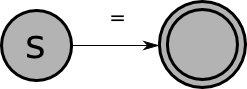ID: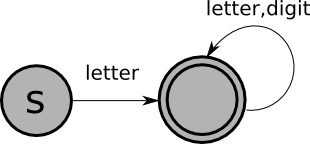PLUS: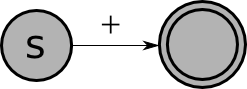TIMES: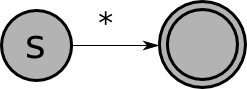EQUALS: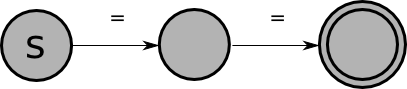The remainder of this section addresses the following problem: Given an FSM for each token, how do we create a scanner?''

## Scanning: Problem Definition

An FSM only checks language membership. That is, given an FSM M, it can answer the question Given a string ω, is ω ∈ L(M)?'' A scanner (a.k.a. a tokenizer) needs more:

• It needs to break up into tokens a stream made up of many different tokens (each defined by its own FSM)
• It needs to successively find the next token by a maximal munch'':
• the longest prefix of the remaining input that corresponds to a token
and return information about what was matched
Thus, the problem definition is as follows:
Given a collection of token definitions (in the form of one FSM for each kind of token), create a maximal-munch tokenizer.

## Method 1

Recall that the goal of a scanner is to find the longest prefix of the current input that corresponds to a token. This has two consequences:

1. The scanner sometimes needs to look one or more characters beyond the last character of the current token, and then needs to "put back" those characters so that the next time the scanner is called it will have the correct current character. For example, when scanning a program written in the simple assignment-statement language defined above, if the input is "==", the scanner should return the EQUALS token, not two ASSIGN tokens. So if the current character is "=", the scanner must look at the next character to see whether it is another "=" (in which case it will return EQUALS), or is some other character (in which case it will put that character back and return ASSIGN).

2. It is no longer correct to run the FSM program until the machine gets stuck or end-of-input is reached, since in general the input will correspond to many tokens, not just a single token.

Furthermore, remember that regular expressions are used both to define tokens and to define things that must be recognized and skipped (like whitespace and comments). In the first case a value (the current token) must be returned when the regular expression is matched, but in the second case the scanner should simply start up again trying to match another regular expression.

With all this in mind, to create a scanner from a set of FSMs, we must:

• modify the machines so that a state can have an associated action to "put back N characters" and/or to "return token XXX",
• we must combine the finite-state machines for all of the tokens in to a single machine, and
• we must write a program for the "combined" machine.

For example, the FSM that recognizes Pascal identifiers must be modified as follows: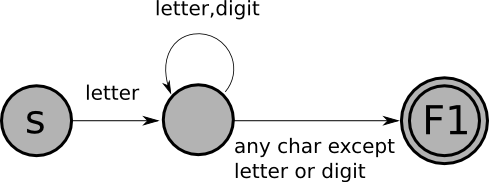with the following table of actions:

Actions:
F1: put back 1 char, return ID

And here is the combined FSM for the five tokens (with the actions noted below):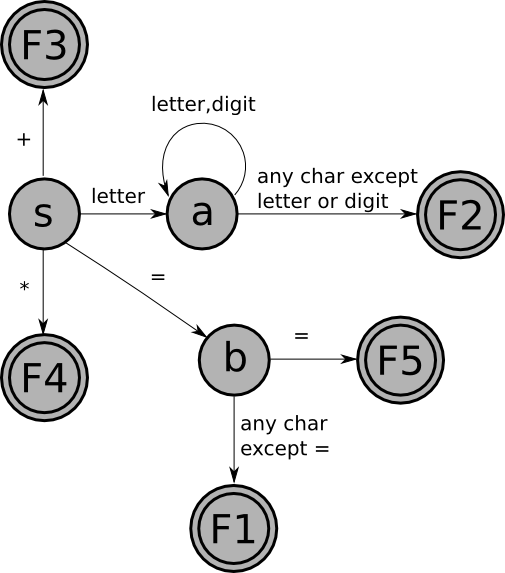with the following table of actions:

Actions:

F1: put back 1 char; return ASSIGN
F2: put back 1 char; return ID
F3: return PLUS
F4: return TIMES
F5: return EQUALS

We can convert this FSM to code using the table-driven technique described above, with a few small modifications:

• The table will include a column for end-of-file as well as for all possible characters (the end-of-file column is needed, for example, so that the scanner works correctly when an identifier is the last token in the input).
• Each table entry may include an action as well as or instead of a new state.
• Instead of repeating "read a character; update the state variable" until the machine gets stuck or the entire input is read, the code will repeat: "read a character; perform the action and/or update the state variable" (eventually, the action will be to return a value, so the scanner code will stop, and will start again in the start state next time it is called).
Here's the table for the above "combined" FSM:

 + * = $\mathrm{letter}$ $\mathrm{digit}$ EOF $S$ return PLUS return TIMES $B$ $A$ $A$ put back 1 char; return ID put back 1 char; return ID put back 1 char; return ID $A$ $A$ return ID $B$ put back 1 char; return ASSIGN put back 1 char; return ASSIGN return EQUALS put back 1 char; return ASSIGN put back 1 char; return ASSIGN return ASSIGN

TEST YOURSELF #3

Suppose we want to extend the very simple language of assignment statements defined above to allow both integer and double literals to occur on the right-hand sides of the assignments. For example:

x = 23 + 5.5
would be a legal assignment.

What new tokens would have to be defined? What are the regular expressions, the finite-state machines, and the modified finite-state machines that define them? How would the the "combined" finite-state machine given above have to be augmented?

solution

## Method 2

Unfortunately, the technique for creating a scanner from a set of FSMs described in Method 1 has some drawbacks. As we saw above, the issue that complicates matters has to do with overlaps in tokens:

• = vs. ==
• + vs. +=
• The keyword for'' vs. the identifier formula''
The scanner must know how to resolve such ambiguities.

In fact, the above examples are all handled correctly by Method 1 (why?), and typically there is no issue for the kind of overlaps that arise in the lexical syntax of a programming language. However, in general, there can be a problem. For instance, consider the following token definitions

 Token Regular Expression TOKEN1 abc TOKEN2 (abc)*d
and the input string abcabcabc''. The desired result is that the input string should be tokenized as TOKEN1 TOKEN1 TOKEN1.

More generally, suppose the input string were of the form (abc)n, where the superscript n'' means that the string has n repetitions of abc''. The desired result is that the input string should be tokenized as TOKEN1n. The problem is that for the scanner to establish that the first three characters should be tokenized as TOKEN1—as opposed to making up the first three characters of a longer TOKEN2—the scanner has to visit all the characters of the input before deciding that there is no final d'' as required for the token to be TOKEN2; consequently, the scanner has to back up 3*(n-1) characters so that the input that remains to be tokenized is (abc)n-1. In this case, the amount of backup is proportional to the length of the string, and hence is unbounded (i.e., not bounded by any fixed constant, independent of n).

The idea behind the tokenization algorithm is as follows:

• Use one DFA for each kind of token (e.g., M1 for abc and M2 for (abc)*d).
• Start running all DFAs simultaneously on the remaining input.
• A DFA drops out when it enters a stuck state (i.e., has no available transition on the next input character).
• Update most_recent_accepted_position and most_recent_accepted_token whenever any machine enters a final state. (Break ties by assigning some precedence order to the DFAs.)
• When the last DFA drops out,
• Return most_recent_accepted_token (or FAIL, if most_recent_accepted_token was never set).
• For finding the next token, the remaining input starts at most_recent_accepted_position.
Using the most-recent accepted position ⇒ the longest token is identified ⇒ a maximal munch'' is performed each time a token is identified.

### Example:

Let's consider again the example in which we have TOKEN1 =def abc; TOKEN2 =def (abc)*d; and the input string is abcabcabc''. (We've specified TOKEN1 and TOKEN2 using regular expressions, but it is easy to give equivalent FSMs for them. Call them M1 and M2, respectively.) Here is a synopsis of what happens when the tokenization algorithm is run:

• The algorithm consumes the first instance of abc.''
• The machines for both TOKEN1 (M1) and TOKEN2 (M2) are still in play.
• M1 is in an accepting state.
• On the next a,'' M1 drops out; M2 is still in play, but it is not in an accepting state.
• After the next bcabc,'' M2 drops out, but it never entered its accepting state.
• TOKEN1 is returned.
• The remaining input (i.e., abcabc) is handled similarly, and two more instances of TOKEN1 are returned.
• The overall result is that abcabcabc'' is tokenized as TOKEN1 TOKEN1 TOKEN1, as desired.

The drawback of the tokenization algorithm is that for an example like the one discussed above, the cost of the algorithm is O(n2). However, it is possible to give a linear-time algorithm for maximal-munch tokenization. See Reps, T., Maximal-munch' tokenization in linear time.'' ACM TOPLAS 20, 2 (March 1998), pp. 259-273.

Here is another variant of the tokenization algorithm, which uses one DFA. Suppose that the tokens are defined by the regular expressions R1, R2, ..., Rk. Let M be a DFA for which L(M) = L(R1 | R2 | ... | Rk).

Notation: We use mrap'' to abbreviate most_recent_accepted_position,'' and mrat'' to abbreviate most_recent_accepted_token.'' We also assume that there is an auxiliary function, tokenFor(q),'' which, for each final state q ∈ F, provides information about what token should be returned when q is the final state corresponding for the most-recent accepted token. It is easy to construct tokenFor(q) during the construction of M, and it is how M accounts for the precedence order among tokens if there is any ambiguity among the token definitions.

Tokenize(M: DFA, input: string)
let [Q, Σ, δ, q0, F] = M in
begin
i = 0;
forever {
q = q0;
mrap = -1;
mrat = -1;
while (i < length(input)) {
q = δ(q, input[i]);
i = i + 1;
if (q ∈ F) {
mrap = i;
mrat = tokenFor(q);
}
}
if (mrap == -1) return 'FAIL'
i = mrap;
print(mrat);
if (i ≥ length(input)) return 'SUCCESS'
}
end`